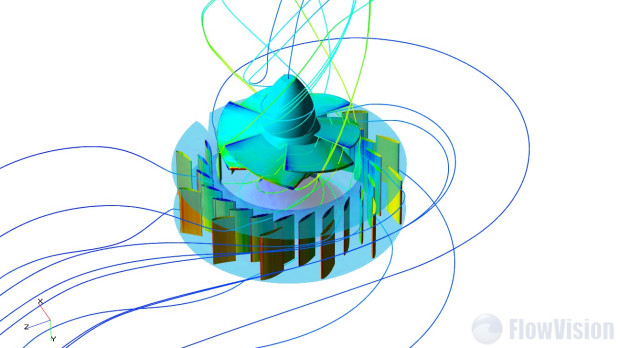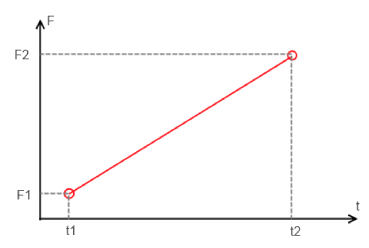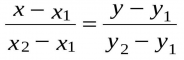Smooth changing of variable value in FlowVision

Too quick changing of variable can give divergence or make convergence worse, because fast changes will give large gradients of physical variables.

For example, in tasks about turbines and compressors we have rotor which has large velocity of rotation. When we start simulation we can’t specify initial velocity for gas between blades of rotor, because structure of flow too complex. It means that during first time step we will have quick changes of velocity from zero (initial velocity of gas) to some large velocity of blades.If we will solve this task for incompressible liquid, we will have divergence. It is possible to make convergence better if we will exclude too quick changes, for example, we can change speed of rotation smoothly from zero.

FlowVision allow to specify very complex equations for any user’s variables. Below you will find some formula template which useful to use every time when you need specify some smooth changing of variable.

Usually we know initial value of variable which we want to use at beginning ( F1 ) and we know value which we want to have at the end (F2). Also we know for what time we want to go from F1 to F2 ( dt ) and we know when we want to start this changing ( t1 ).

In this case we can use equation of straight line which determined by to plots:Template for linear changing variable

//Specify what variable will determine time dependence. It can be number of step or current time.
t=StepNumber;

//What is initial value of variable and what is final value
F1=1000;
F2=11768400;

//t1 – it is time when our variable will start changing
t1=625;

//Usually I prefer specify time interval (dt) instead time (t2) when variable F must be = F2 (finishing of changing)
dt=50;

//t2  will be calculated automatically :
t2=t1+dt;

//Calculation of variable F. If t<t1 then F=F1, if t>t2 then F=F2,
//between t1 and t2 we use liner law changing of F:

{

(t<t1): F1;
(t>t2): F2;
( (t>=t1) AND (t<=t2) ): (t-t1)/(t2-t1)*(F2-F1)+F1;

}

With same way you can create template for square-law.